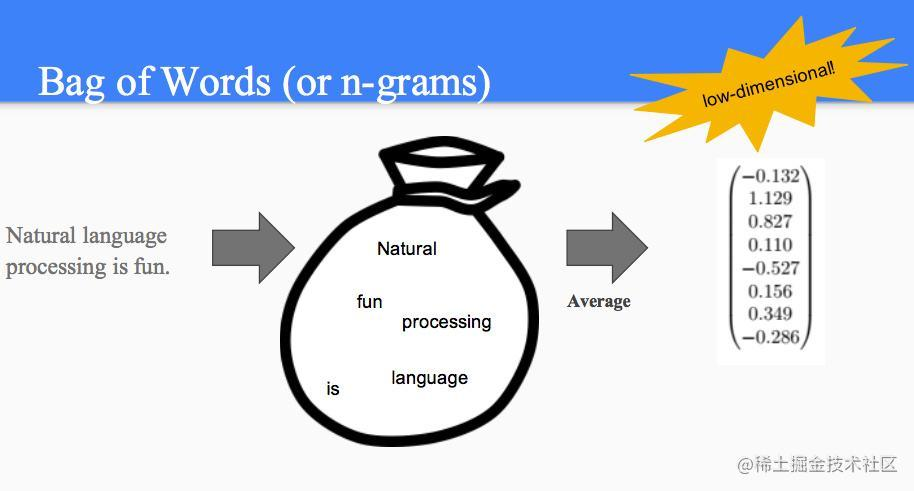# 教你如何從0-1使用機器學習利器Sklearn實現文字分類企業級案例

### 前言### 正文

##### 載入資料集

filepath_dict = {'yelp': 'data/sentiment_analysis/yelp_labelled.txt', 'amazon': 'data/sentiment_analysis/amazon_cells_labelled.txt', 'imdb': 'data/sentiment_analysis/imdb_labelled.txt'}

df_list = [] for source, filepath in filepath_dict.items(): df = pd.read_csv(filepath, names=['sentence', 'label'], sep='t') df['source'] = source # Add another column filled with the source name df_list.append(df)

df = pd.concat(df_list) print(df.iloc) ```

##### 文字轉特徵資料 CountVectorizer

vectorizer = CountVectorizer(min_df=0, lowercase=False) vectorizer.fit(sentences) print(vectorizer.vocabulary_) print(vectorizer.transform(sentences).toarray()) ```

##### 資料集切分 train_test_split

df_yelp = df[df['source'] == 'yelp']

sentences = df_yelp['sentence'].values y = df_yelp['label'].values

sentences_train, sentences_test, y_train, y_test = train_test_split(sentences, y, test_size=0.25, random_state=1000) ```

##### 基於詞袋模型分類

classifier = LogisticRegression() classifier.fit(X_train, y_train) score = classifier.score(X_test, y_test)

print("Accuracy:", score)

for source in df['source'].unique(): df_source = df[df['source'] == source] sentences = df_source['sentence'].values y = df_source['label'].values

``````sentences_train, sentences_test, y_train, y_test = train_test_split(
sentences, y, test_size=0.25, random_state=1000)

vectorizer = CountVectorizer()
vectorizer.fit(sentences_train)
X_train = vectorizer.transform(sentences_train)
X_test = vectorizer.transform(sentences_test)

classifier = LogisticRegression()
classifier.fit(X_train, y_train)
score = classifier.score(X_test, y_test)
print('Accuracy for {} data: {:.4f}'.format(source, score))
``````

``` 好啦，這樣就可以了。趕緊試試吧！